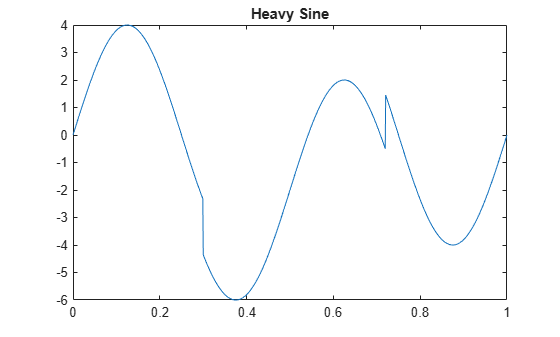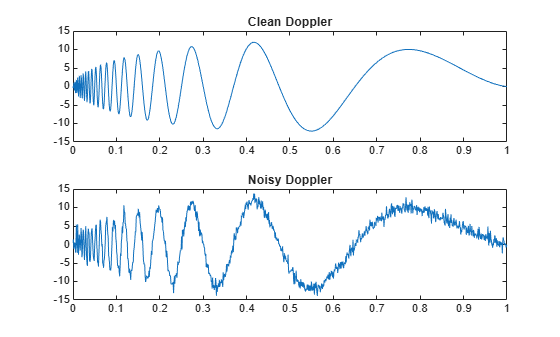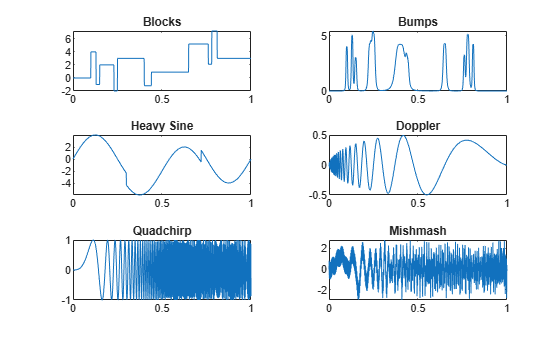# wnoise

Noisy wavelet test data

## Syntax

``x = wnoise(fun,n)``
``[x,xn] = wnoise(fun,n,sqrtsnr)``
``[x,xn] = wnoise(___,init)``

## Description

example

````x = wnoise(fun,n)` returns values `x` of the test signal `fun` evaluated at 2n linearly spaced points from 0 to 1.```
````[x,xn] = wnoise(fun,n,sqrtsnr)` returns `x` rescaled such that the standard deviation of x is `sqrtsnr`. `xn` is `x` corrupted by additive Gaussian white noise N(0,1) and has a signal-to-noise ratio (SNR) of `sqrtsnr`2.```
````[x,xn] = wnoise(___,init)` sets the generator seed to `init` before generating additive Gaussian white noise N(0,1) .```

## Examples

collapse all

There are six test signals. Generate and plot ${2}^{10}$ samples of the third test signal, `heavy sine`.

```loc = linspace(0,1,2^10); x = wnoise(3,10); plot(loc,x) title('Heavy Sine')```Generate and plot ${2}^{10}$ samples of the `doppler` test signal and a noisy version of `doppler` with a square root of the signal-to-noise ratio equal to 7.

```[x,noisyx] = wnoise('doppler',10,7); subplot(2,1,1) plot(loc,x) title('Clean Doppler') ylim([-15 15]) subplot(2,1,2) plot(loc,noisyx) title('Noisy Doppler') ylim([-15 15])```Plot all the test functions.

```testFunctions = {'Blocks','Bumps','Heavy Sine','Doppler','Quadchirp','Mishmash'}; for i=1:6 x = wnoise(lower(testFunctions{i}),10); subplot(3,2,i) plot(loc,x) title(testFunctions{i}) end```## Input Arguments

collapse all

Wavelet test function, specified as one of the values listed here. The six test functions are due to Donoho and Johnstone , .

• 1 or `'blocks'`

• 2 or `'bumps'`

• 3 or `'heavy sine'`

• 4 or `'doppler'`

• 5 or `'quadchirp'`

• 6 or `'mishmash'`

Exponent used to determine the number of linearly spaced points from 0 to 1 to evaluate the test function, specified as a positive integer. The number of linearly spaced points is 2n.

Square root of SNR, specified by a positive real number. The test values `x` are rescaled such that the standard deviation of `x` is `sqrtsnr`. `xn` is equal to `x` corrupted by additive Gaussian white noise N(0,1) and has an SNR of `sqrtsnr`2.

Seed used to initialize the random number generator, specified as a nonnegative integer. `init` is used to generate additive Gaussian white noise.

Example: `[a,b] = wnoise(4,10,7,2055415866);` returns a noisy version of the fourth test signal using the seed ```init = 2055415866```.

## Output Arguments

collapse all

Test signal, returned as a real-valued vector of length 2`n`. `x` are the values of the test function specified by `fun` evaluated at the 2`n` evenly spaced points from 0 to 1. If `sqrtsnr` is set, the standard deviation of `x` is `sqrtsnr`.

Noisy test signal, returned as a real-valued vector of length 2`n`. `xn` is `x` corrupted by additive Gaussian white noise N(0,1) and has an SNR of `sqrtsnr`2.

 Donoho, D. L., and I. M. Johnstone. “Ideal spatial adaptation by wavelet shrinkage.” Biometrika. Vol. 81, Issue 3, 1994, pp. 425–455.

 Donoho, D. L., and I. M. Johnstone. “Adapting to unknown smoothness via wavelet shrinkage.” Journal of the American Statistical Association. Vol. 90, 1995, pp. 1200–1224.